Next: The solution space of Up: Graphical solution of 2-var Previous: Feasible Regions of Two-Var

#### The solution space of a single equality constraint

We start our investigation regarding the geometrical representation of 2-var linear constraints by considering first constraints of the equality type, i.e.,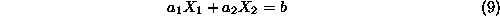It is a well-known result that, assuming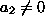, this equation corresponds to a straight line with slope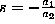and intercept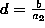. In the special case where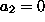, the solution space (locus) of Equation 9 is still a straight line perpendicular to the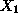-axis, intersecting it at the point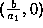. Notice that the presence of an equality constraint restricts the dimensionality of the feasible solution space by one degree of freedom, i.e., it turns it from a planar area to a line segment.

UAL Data
Fri Jun 20 15:03:05 CDT 1997